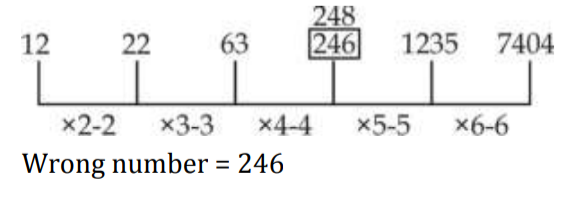# gkrecall

We brings you daily Current affairs, daily Current Affairs Quiz, weekly Current Affairs, weekly Current Affairs Quiz to enhance your preparation for upcoming exams. We also provide articles related to quant, reasoning, English, ssc etc .

## Breaking

We bring you the complete and important daily QUANT  to achieve more marks in Banking, Insurance, UPSC, SSC, CLAT, Railways and all other competitive Exams. We prepare it based on our daily current affairs.Hope you like it.START TEST

Directions (1-15): Find the wrong term which does not follow the pattern that other numbers follow
in the following number series:
Q1. 28, 66, 128, 224, 348, 518
(a) 348
(b) 224
(c) 66
(d) 518
(e) 28
Q2. 12, 22, 63, 246, 1235, 7404
(a) 246
(b) 7404
(c) 12
(d) 63
(e) 1235
Q3. 1634, 905, 562, 439, 410, 409
(a) 439
(b) 410
(c) 409
(d) 1634
(e) 905
Q4. 5, 25, 129, 780, 5441, 43529
(a) 5441
(b) 129
(c) 25
(d) 780
(e) 5
Q5. 16, 40, 100, 250, 630, 1562.5
(a) 630
(b) 40
(c) 16
(d) 100
(e) 250

Directions (11-15): In the given question, two equations numbered I and II are given. You have to solve both the equations andGive answer:
(a) If x < y
(b) If x > y
(c) If x ≥ y
(d) If x ≤ y
(e) If relationship between x and y cannot be established.
Q11. I. 2x² - 7x+ 3 = 0
II. 2y² - 7y + 6 = 0

Q12. I. 4x² + 16x +15 = 0
II. 2y² + 3y + 1 = 0

Q13. I. 9x² - 45x + 56 = 0
II. 4y² - 17y + 18 = 0

Q14. I. 2x² + 11x + 14 = 0
II. 2y² + 15y + 28 = 0

Q15. I. 6x² + 11x + 4 = 0
II. 4y² - 7y – 2 = 0

SOLUTION

S1. Ans.(b)
Sol. Pattern is
3³ + 1 = 28
4³ + 2 = 66
5³+ 3 = 128
6³ + 4 = 220
7³ + 5 = 348
8³ + 6= 518
∴ Wrong term = 224

S2. Ans.(a)
Sol.
Wrong number = 246S3. Ans.(a)
Sol. Pattern is
–9³, –7³, –5³, –3³, –1³
∴ Wrong term = 439

S4. Ans.(d)
Sol. Pattern is
×4 + 5, ×5+ 4, × 6 + 3, × 7+2, ×8 +1
∴ Wrong term = 780

S5. Ans.(a)
Sol. Pattern is
16 × 2.5 = 40
40 × 2.5 = 100
100 × 2.5 = 250
250 × 2.5 = 625
625 × 2.5 = 1562.5
So, 630 is wrong term

S11. Ans.(e)
Sol.
I. 2x² - 7x+ 3 =0
⇒ 2x² - 6x – x + 3 = 0
⇒ (x- 3) (2x- 1) = 0
x =3, 1/2

II. 2𝑦
2 − 7𝑦 + 6 = 0
⇒ 2y² - 4y -3y+ 6 = 0
⇒ (y- 2) (2y - 3) = 0
⇒ 𝑦 = 2,
3/2

No relation between x & y
S12. Ans.(a)
Sol.
I. 4𝑥
2 + 16𝑥 + 15 = 0
⇒ 4𝑥
2 + 10𝑥 + 6𝑥 + 15 = 0
⇒ (2x + 5) (2x + 3) = 0
⇒ 𝑥 =
,-5/2
−3/2
II. 2𝑦
2 + 3𝑦 + 1 = 0
⇒ (2y+ 1) (y+ 1) = 0
⇒ 𝑦 = −1, −1/2
y>x

S13. Ans.(b)
Sol.
I. 9x² - 45x + 56 = 0
⇒ 9x² - 21x – 24x + 56 = 0
⇒ (3x – 7) (3x- 8) = 0
⇒ 𝑥 =7/3,8/3

II. 4𝑦
2 − 17𝑦 + 18 = 0
⇒ 4y² - 8y – 9y + 18 = 0
⇒ (y- 2) (4y- 9) = 0
⇒ y= 2, 9/4
x >y

S14. Ans.(c)
Sol.
I. 2x² + 11x + 14 = 0
⇒ 2x² + 4x+ 7x+ 14 = 0
⇒ (x+ 2) (2x+ 7) = 0
⇒ x = -2, -7/2
II. 2y² + 15y + 28 = 0
⇒ 2y² + 8y+ 7y+ 28 = 0
⇒ (y+ 4) (2y+ 7) = 0
⇒ y = -4, −7/2
x ≥ y

S15. Ans.(a)
Sol.
I. 6x² + 11x+ 4 = 0
⇒ 6x² +8x+ 3x+ 4 = 0
⇒ (3x+ 4) (2x+ 1) = 0
⇒ x = −4/3
, −1/2
II. 4𝑦
2 − 7𝑦 − 2 = 0
⇒ 4y² - 8y +y – 2 = 0
⇒ (y – 2) (4y+ 1) = 0
⇒ 𝑦 = 2, −1/4
y > x